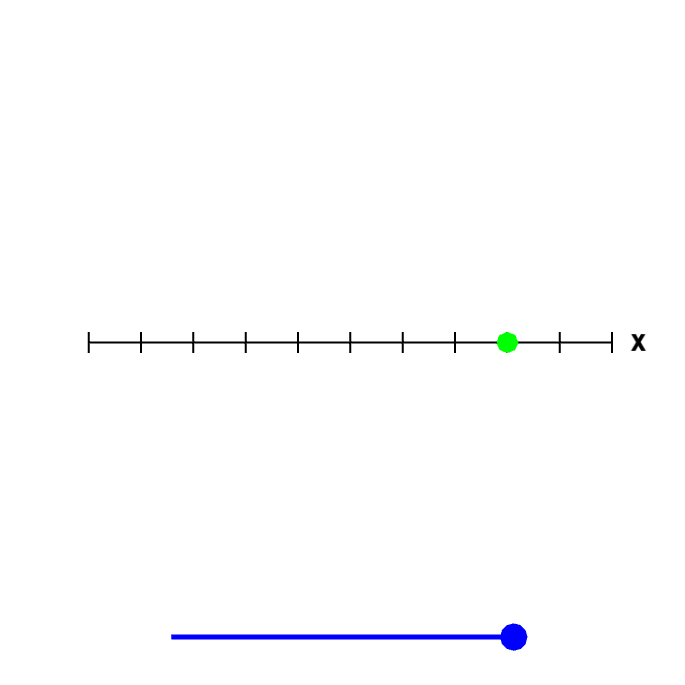# Math Insight

### Applet: A point or a lineThe graph of the equation $x=3$ could be a point, if it were an equation in one variable. But, in this case, it is an equation in two dimensions, in the variables $x$ and $y$ (where the coefficient of $y$ just happens to be zero). Rotate the figure by moving the blue point on the slider to the left to see that, since it is a graph in two dimensions, the graph is really a line.